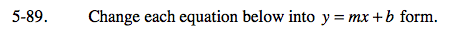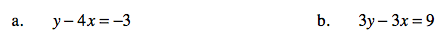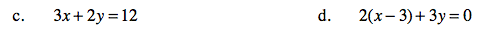### Home > CAAC > Chapter 5 > Lesson 5.2.3 > Problem5-89

5-89.Solve the equation for y.

y − 4x = −3
+4x +4x

y = 4x − 3

Solve the equation for y.

3y − 3x = 9
+3x +3x

3y = 3x + 9

y = x + 3Follow the steps in part (b).

First distribute the 2.

Follow the steps in part (b).

$y=-\frac{2}{3}{x}+2$